# 5. The Pi-theorem.

Theorem: Letbe a unit free physical law that relates the dimensioned quantities q1,q2,…,qm. Let L1,L2,…,Ln (where n<m) be the fundamental dimensions with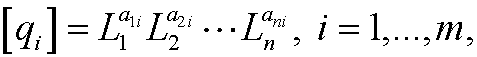and let r=rank(A), where A is the dimension matrix. Then there are m-r independent dimensionless quantitiesthat can be formed from q1,q2,…,qm. Moreover, the physical law above is equivalent with an equation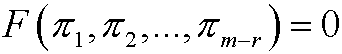which is solely expressed in dimensionless quantities.

Example: In our introductory example we had(m=4) with the fundamental dimensions T, L, M (n=3) andThe dimension matrix will then becomewith rank(A)=3. This implies that we have m-r=4-3=1 dimensionless variable.

Remark: The following three characterisations of the rank rank(A) of a matrix A are equivalent:
1) The number of nonzero rows of the transformed upper triangular matrix.
2) The number of linearly independent rows or columns of A.
3) The highest order of nonzero subdeterminants to A.

Example: The matrix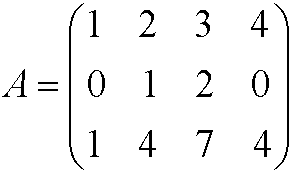has rank 2.
Method 1 gives rank(A)=2 since admissible row operations show thatMethod 2 gives rank(A)=2 since row3 is row1 plus 2 times row2 while row2 is not a multiple of row1

Method 3 gives rank(A)=2 sincewhileProof (not complete) of the Pi-theorem:
Letbe the dimensionless quantity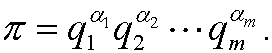Expressed in the fundamental quantities L1,L2,,…,Ln we then have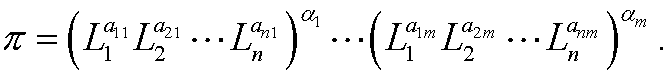Sincethe exponents must be 0, that is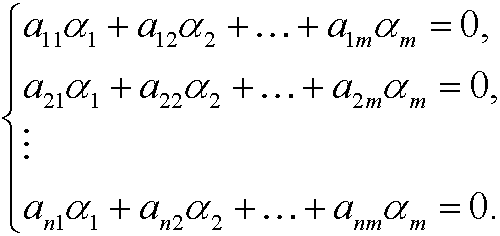We have m unknowns and n equations, where m>n and the system rank is r. From linear algebra we know that there are m-r linearly independent solutions. Every such solution induces one dimensionless variable.